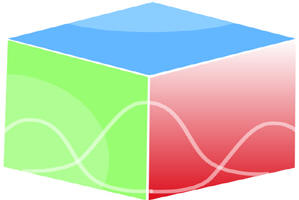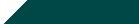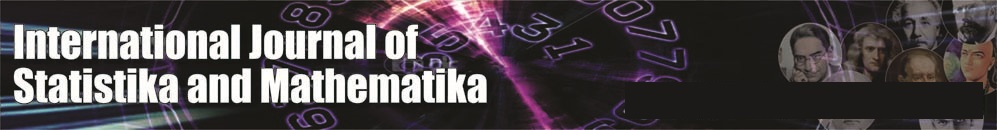``Home| Journals | Statistics Online Expert | About Us | Contact UsUntitled Document

[

Gain-function of two non-identical warm standby system with failure due to non- availability of wind and switch failure
Ashok Kumar Saini

Associate Professor, Department of Mathematics, B. L. J. S. College, Tosham, Bhiwani, Haryana, INDIA.

Email: [email protected]

Research Article

Abstract               Introduction: The worldwide installed capacity of wind power reached 283 GW by the end of 2012. China (75,564 MW), US (60,007 MW), Germany (31,332 MW) and Spain (22,796 MW) are ahead of India in fifth position. The short gestation periods for installing wind turbines, and the increasing reliability and performance of wind energy machines has made wind power a favoured choice for capacity addition in India. In Wind turbines, the wind plays an important and vital role. The non-conventional renewable wind energy which is cheap and readily available for use to produce electricity for institutions, hospitals, industries and upliftment of water to higher places for agriculture. Wind is the prime source from where wind energy can be generated. But when wind is not blowing strongly the wind turbines are unable to receive wind which causing failure of the system. In the present paper we have taken two non-identical warm standby system with failure due to non- availability of wind. When there is non-availability of wind the working of unit stops automatically. The failure time distribution is taken as exponential and repair time distribution as general. Using Semi Markov regenerative point technique we have calculated different reliability characteristics such as MTSF, reliability of the system, availability analysis in steady state, busy period analysis of the system under repair, expected number of visits by the repairman in the long run and profit-function. Special case by taking repair as exponential has been derived and graphs are drawn.

Keywords: warm standby, non-availability of wind, MTSF, Availability, busy period, Gain-function.

INTRODUCTION

The development of wind power in India began in the 1990s, and has significantly increased in the last few years. Although a relative newcomer to the wind industry compared with Denmark or the United States, India has the fifth largest installed wind power capacity in the world. In 2009-10 India's growth rate was highest among the other top four countries. As of 31 March 2014 the installed capacity of wind power in India was 21136.3 MW. Wind power accounts for 8.5% of India's total installed power capacity, and it generates 1.6% of the country's power. To generate Wind –energy the wind plays the pivotal and vital role. The non-conventional renewable wind energy which is cheap and easily available for use produce wind energy which turn the large wind panes of wind-turbines to produce electricity for institutions, hospitals, industries, and upliftment of water for drinking and irrigation for cities and agriculture. Wind is the primary source from where wind energy can be generated. When wind turbines becomes ineffective due to non-availability of wind resulting failure of the system.

Assumptions

1. The failure time distribution is exponential whereas the repair time distribution is arbitrary of two non-identical units.
2. The repairs are perfect and starts immediately upon failure of units with repair discipline are FCFS.
3. The operation of the unit stops as soon as there is non-availability of wind.
4. The failure of a unit is detected immediately and perfectly.
5. The switches are instantaneous but not perfect.
6. All random variables are mutually independent.

SYMBOLS FOR STATES OF THE SYSTEM

Superscripts: O, WS, SO, FNAW, SF

Operative, Warm Standby, Stops the operation, Failure due to non-availability of wind, Switch failure respectively

Subscripts: nasl, asl, ur, wr, uR

Non-availability of wind, availability of wind, under repair, waiting for repair, under repair continued respectively

Up states: 0, 1, 2, 9;

Down states: 3, 4, 5, 6, 7, 8, 10, 11

Regeneration point: 0, 1, 2, 4, 7, 10

States of the System

0(Oasl, WSnasl)

The first unit is operative due to availability of wind and the second unit is warm standby with non-availability of wind.

1(SOnasl, Oasl)

The operation of the first unit stops automatically due to non-availability of wind and warm standby units starts operating due to availability of wind.

2(FNASLur, Oasl)

The first unit fails due to non-availability of wind undergoes repair and the second unit continues to be operative due to availability of wind.

3(FNASLuR, SOnasl)

The repair of the first unit is continued from state 2 and in the other unit the operation of the unit stops automatically due to non-availability of wind.

4(FNASLur, SOnasl)

The one unit fails due to non-availability of wind and undergoes repair and the other unit also stops automatically due to non-availability of wind.

5(FNASLuR, FNASLwr)

The repair of the first unit is continued from state 4 and the other unit is failed due to non-availability of wind in it and is waiting for repair.

Figure 1: The State Transition Diagram

6 (Oasl,FNASLur)

The first unit is operative due to availability of wind and the second unit failed due to non-availability of wind is under repair.

7(SOnasl,SFur)

The operation of the first unit stops automatically due to non-availability of wind and during switchover to the second unit switch fails and undergoes repair.

8(FNASLwr,SFuR)

The repair of failed switch is continued from state 7 and the first unit is failed due to non-availability of wind is waiting for repair.

9(Oasl,SOnasl)

The first unit is operative due to availability of wind and the operation of warm standby second unit is stopped due to non-availability of wind.

10(SOnasl,SFur)

The operation of the first unit stops automatically due to non-availability of wind and in the second unit switch fails and undergoes repair.

11(FNASLwr,SFuR)

The repair of the second unit is continued from state 10 and the first unit is failed due to non-availability of wind is waiting for repair.

TRANSITION PROBABILITIES

Simple probabilistic considerations yield the following expressions:

p01 = ,P07 =

p09 = ,p12 = ,p14 =

P20= G1*( λ1),P22(3) = G1*( λ1)=p23,P72 = G2*( λ4),

P72(8) = G2*( λ4)= P78

We can easily verify that

p01 + p07 + p09 = 1, p12 + p14 = 1, p20 + p23 (=p22(3))= 1,p46(6)= 1 p60 = 1,

p72+ P72(5) + p74 = 1,p9,10 =1, p10,2 + p10,2(11) = 1                                                                                                                       (1)

We can easily verify that

p01 + p07 + p09 = 1, p12 + p14 = 1, p20 + p23 (=p22(3))= 1,p46(6)= 1 p60 = 1,

p72+ P72(5) + p74 = 1,p9,10 =1, p10,2 + p10,2(11) = 1 (1)

And mean sojourn time are

µ0 = E(T) =                                                                                                                                                              (2)

Mean Time to System Failure

We can regard the failed state as absorbing

,

(3-5)

Taking Laplace-Stiltjes transform of eq. (3-5) and solving for

= N1(s) / D1(s)                                                                                                                                                                   (6)

Where

N1(s) = {  +

D1(s) = 1 -

Making use of relations (1) and (2) it can be shown that θ0(0) =1,which implies that θ0(t) is a proper distribution.

MTSF = E[T] = d/ds θ0*(0)= (D1(0) - N1(0)) / D1 (0)

s=0

= ( +p01  + p01 p12  + p09 ) / (1 - p01 p12 p20 )

where

+  + , + , + (3),

AVAILABILITY ANALYSIS

Let Mi(t) be the probability of the system having started from state I is up at time t without making any other regenerative state belonging to E. By probabilistic arguments, we have

The value of M0 (t), M1 (t), M2 (t), M4 (t) can be found easily.

The point wise availability Ai (t) has the following recursive relations

A0(t) = M0(t) + q01(t)[c]A1(t) + q07(t)[c]A7(t) + q09(t)[c]A9(t)

A1(t) = M1(t) + q12(t)[c]A2(t) + q14(t)[c]A4(t),A2(t) = M2(t) + q20(t)[c]A0(t) + q22(3)(t)[c]A2(t)

A4(t) = q46(3)(t)[c]A6(t),A6(t) = q60(t)[c]A0(t)

A7(t) = (q72(t)+ q72(8)(t)) [c]A2(t) + q74 (t)[c]A4(t)

A9(t) = M9(t) + q9,10(t)[c]A10(t),A10(t) = q10,2(t)[c]A2(t) + q10,2(11)(t)[c]A2(t)                                                                              (7-14)

Taking Laplace Transform of eq. (7-14) and solving for

= N2(s) / D2(s)                                                                                                                                                                  (15)

where

N2(s) = (1 -  22(3)(s)) {  0(s) + 01(s) 1(s) + 09(s) 9(s)}+ 2(s){ 01(s) 42(s) + 07(s)72(s) +  73(8)(s)) +  09

(s) 9,10 (s)( 10,2 (s) + 10,2(11)(s))}

D2(s) = (1 -  22(3)(s)) { 1 -  46(5)(s) 60(s) ( 01(s) 44 (s) + 07(s) 74(s))

- 20(s)01(s)12(s)07(s)(  72(s)) +  72(8)(s) +  09 (s) 9,10 (s)

( 10,2 (s) + 10,2(11)(s))}

A0 =  =  =

Using L’ Hospitals rule, we get

A0 =  =                                                                                                                                           (16)

Where

N2(0)= p20(0(0) + p011(0) + p09 9(0) ) + 2(0) (p01p12 + p07 (p72

+ p72(8) + p09 ))

D2(0) = p20{  + p01 + (p01 p14 + p07 p74 )+ p07  + p07  + p09()

+ { 1- ((p01p14 + p07 p74 )}

,,

The expected up time of the system in (0, t] is

(t) =  So that                                                                                                       (17)

The expected down time of the system in (0,t] is

(t) = t- (t) So that                                                                                                                   (18)

The expected busy period of the server for repairing the failed unit under non-availability of wind in (0, t]

R0(t) = S0(t) + q01(t)[c]R1(t) + q07(t)[c]R7(t) + q09(t)[c]R9(t)

R1(t) = S1(t) + q12(t)[c]R2(t) + q14(t)[c]R4(t),

R2(t) = q20(t)[c]R0(t) + q22(3)(t)[c]R2(t)

R4(t) = q46(3)(t)[c]R6(t),R6(t) = q60(t)[c]R0(t)

R7(t) = (q72(t)+ q72(8)(t)) [c]R2(t) + q74 (t)[c]R4(t)

R9(t) = S9(t) + q9,10(t)[c]R10(t),R10(t) = q10,2(t) + q10,2(11)(t)[c]R2(t)                                                                                                (19-26)

Taking Laplace Transform of eq. (19-26) and solving for

= N3(s) / D2(s) (27)

Where

N2(s) = (1 -  22(3)(s)) {  0(s) + 01(s) 1(s) + 09(s) 9(s)} and D2(s) is already defined.

In the long run, R0 =                                                                                                                                                        (28)

where N3(0)= p20(0(0) + p011(0) + p09 9(0) ) and D2(0) is already defined.

The expected period of the system under non-availability of wind in (0, t] is

(t) =  So that

The expected Busy period of the server for repair of dissimilar units by the repairman in (0, t]

B0(t) = q01(t)[c]B1(t) + q07(t)[c]B7(t) + q09(t)[c]B9(t)

B1(t) = q12(t)[c]B2(t) + q14(t)[c]B4(t),B2(t) = q20(t)[c] B0(t) + q22(3)(t)[c]B2(t)

B4(t) = T4 (t)+ q46(3)(t)[c]B6(t),B6(t) = T6 (t)+ q60(t)[c]B0(t)

B7(t) = (q72(t)+ q72(8)(t)) [c]B2(t) + q74 (t)[c]B4(t)

B9(t) = q9,10(t)[c]B10(t),B10(t) = T10 (t)+ (q10,2(t) + q10,2(11)(t)[c]B2(t)                                                                                              (29- 36)

Taking Laplace Transform of eq. (29-36) and solving for

= N4(s) / D2(s)                                                                                                                                                                   (37)

where

N4(s) = (1 -  22(3)(s)) { 01(s)14(s) 4(s) + 46 (5)(s) 6(s)) + 07(3)(s) 74(s)( 4(s)

+  46(5)(s) 6(s))+ 09(s)09,10(s)  10(s) )

In steady state, B0 =                                                                                                                                                       (38)

where N4(0)= p20 {( p01 p14 + p07 p74) (4(0) +6(0)) + p09 10(0) } and D2(0) is already defined.

The expected busy period of the server for repair in (0, t] is

(t) =  So that                                                                                                                      (39)

The expected Busy period of the server for repair of switch in (o, t]

P0(t) = q01(t)[c]P1(t) + q07(t)[c]P7(t) + q09(t)[c]P9(t)

P1(t) = q12(t)[c]P2(t) + q14(t)[c]P4(t),P2(t) = q20(t)[c]P0(t) + q22(3)(t)[c]P2(t)

P4(t) = q46(3)(t)[c]P6(t),P6(t) = q60(t)[c]P0(t)

P7(t) = L7(t)+ (q72(t)+ q72(8)(t)) [c]P2(t) + q74 (t)[c]P4(t)

P9(t) = q9,10(t)[c]P10(t),P10(t) = (q10,2(t) + q10,2(11)(t))[c]P2(t)                                                                                                           (40-47)

Taking Laplace Transform of eq. (40-47) and solving for

= N5(s) / D2(s)                                                                                                                                                                  (48)

here N2(s) = 07(s )  7(s) 1 -  22(3)(s)) and D2(s) is defined earlier.

In the long run, P0 =                                                                                                                                                        (49)

where

N5 (0) = p20 p07 4(0)

The expected busy period of the server for repair of the switch in (0, t] is

(t) =  So that                                                                                                                        (50)

The expected number of visits by the repairman for repairing the non-identical units in (0, t]

H0(t) = Q01(t)[c]H1(t) + Q07(t)[c]H7(t) + Q09(t)[c]H9(t)

H1(t) = Q12(t)[c][1+H2(t)] + Q14(t)[c][1+H4(t)],H2(t) = Q20(t)[c]H0(t) + Q22(3)(t)[c]H2(t)

H4(t) = Q46(3)(t)[c]H6(t),H6(t) = Q60(t)[c]H0(t)

H7(t) = (Q72(t)+ Q72(8)(t)) [c]H2(t) + Q74 (t)[c]H4(t)

H9(t) = Q9,10(t)[c][1+H10(t)],H10(t) = (Q10,2(t)[c] + Q10,2(11)(t))[c]H2(t)                                                                          (51-58)

Taking Laplace Transform of eq. (51-58) and solving for

= N6(s) / D3(s)                                                                                                                                                                   (59)

Where

N6(s) = (1 –  22(3)*(s)) { 01(s)12(s)14(s))  09 (s) 9,10 (s)}

D3(s) = (1 -  22(3)*(s)) { 1 - (01(s) 14 (s) + 07(s) 74(s))46(5)*(s) 60(s)}

- 20(s)01(s)12(s)07(s)( 72(s)) +  72(8)(s) +

09 (s)9,10 (s) ( 10,2 (s) +Q 10,2(11)*(s))}

In the long run, H0 =                                                                                                                                                       (60)

where N6(0)= p20 (p01 + p09) and D’3(0) is already defined.

The expected number of visits by the repairman for repairing the switch in (0, t]

V0(t) = Q01(t)[c]V1(t) + Q07(t)[c]V7(t) + Q09(t)[c]V9(t)

V1(t) = Q12(t)[c]V2(t) + Q14(t)[c]V4(t),V2(t) = Q20(t)[c]V0(t) + Q22(3)(t)[c]V2(t)

V4(t) = Q46(3)(t)[c]V6(t),V6(t) = Q60(t)[c]V0(t)

V7(t) = (Q72(t)[1+V2(t)]+ Q72(8)(t)) [c]V2(t) + Q74 (t)[c]V4(t)

V9(t) = Q9,10(t)[c]V10(t),V10(t) = (Q10,2(t) + Q10,2(11)(t))[c]V2(t)                                                                                       (61-68)

Taking Laplace-Stieltjes transform of eq. (61-68) and solving for

= N7(s) / D4(s)                                                                                                                                                                  (69)

where N7(s) =  07 (s) 72 (s) (1 –  22(3)*(s)) and D4(s) is the same as D3(s)

In the long run, V0 =                                                                                                                                                       (70)

where N7(0)= p20 p07 p72 and D’3(0) is already defined.

GAIN-FUNCTION ANALYSIS

The Gain- function of the system considering mean up-time, expected busy period of the system under non-availability of wind when the units stops automatically, expected busy period of the server for repair of unit and switch, expected number of visits by the repairman for non-identical units failure, expected number of visits by the repairman for switch failure.

The expected total Gain-function incurred in (0, t] is

C (t) = Expected total revenue in (0, t] - expected total repair cost for switch in (0, t]

• expected total repair cost for repairing the units in (0, t]
• expected busy period of the system under non-availability of wind when the units automatically stop in (0, t]
• expected number of visits by the repairman for repairing the switch in (0, t]
• expected number of visits by the repairman for repairing of the non-identical units in (0, t]

The expected total cost per unit time in steady state is

C = =

= K1A0 - K2P0 - K3B0 - K4R0 - K5V0 - K6H0

Where

K1: revenue per unit up-time,

K2: cost per unit time for which the system is under switch repair

K3: cost per unit time for which the system is under unit repair

K4: when units automatically stop cost per unit time for which the system is under non-availability of wind

K5: cost per visit by the repairman for which switch repair,

K6: cost per visit by the repairman for non-identical units repair.

CONCLUSION

After studying the system, we have analyzed graphically that when the failure rate due to non-availability of wind and failure rate due to switch failure increases, the MTSF and steady state availability decreases and the Gain-Function also decreased as the failure increases.

REFERENCES

1. Barlow, R.E. and Proschan, F., Mathematical theory of Reliability, 1965; John Wiley, New York.
2. Gnedanke, B.V., Belyayar, Yu.K. and Soloyer,A.D.,Mathematical Methods of Relability Theory, 1969 ; Academic Press, New York.
3. Dhillon, B.S. and Natesen, J, Stochastic Anaysis of outdoor Power Systems in fluctuating environment, Microelectron. Reliab.. 1983; 23, 867-881.
4. Nakagawa, T., A 2-Unit Repairable Redundant System with Switching Failure, IEEE, Trans. Reliab., 1977; R-26, 2, pp.128-130.
5. Goel, L.R., Sharma, G.C. and Gupta, Rakesh Cost Analysis of a Two-Unit standby system with different weather conditions, Microelectron. Reliab., 1985; 25, 665-659.
6. Goel,L.R., Sharma G.C. and Gupta Parveen,Stochastic Behaviour and Profit Anaysis of a redundant system with slow switching device, Microelectron Reliab., 1986; 26, 215-219.
7. Gupta, Rakesh and Goel, Rakesh, Profit Analysis of a Two-Unit Cold Standby system under Different Weather Conditions, Microelectron. Reliab., 1991;31, pp.18-27.